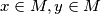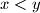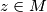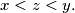### IMO Shortlist 1982 problem 19

Kvaliteta:
Avg: 0.0
Težina:
Avg: 0.0
Let$M$ be the set of real numbers of the form$\frac{m+n}{\sqrt{m^2+n^2}}$, where$m$ and$n$ are positive integers. Prove that for every pair$x \in M, y \in M$ with$x < y$, there exists an element$z \in M$ such that$x < z < y.$
Source: Međunarodna matematička olimpijada, shortlist 1982